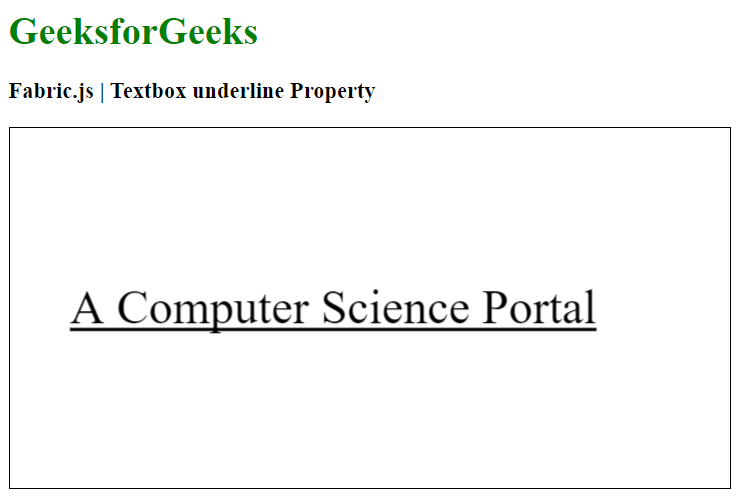# Fabric.js Textbox underline Property

• Last Updated : 22 Jan, 2021

In this article, we are going to see how to add an underline to the canvas-like Textbox using the underline Property of FabricJS. The canvas means Textbox written is movable, rotatable, resizable, and can be stretched. But in this article, we will add an underline to the Textbox. Further, the text itself cannot be edited like a textbox.

Approach: To make it possible we are going to use a JavaScript library called FabricJS. After importing the library using CDN, we will create a canvas block in the body tag which will contain our Textbox. After this, we will initialize instances of Canvas and Textbox provided by FabricJS and use the underline property to add underline to the text and render the Canvas on the Textbox as given in the example below.

Syntax:

```fabric.Textbox('text', {
underline: boolean
});```

Parameters: This function accepts one parameter as mentioned above and described below:

• underline: It specifies whether to add underline or not.

Example: This example uses FabricJS Textbox underline Property to add an underline to the canvas textbox as given below.

## HTML

 ```<``html``>`` ` `<``head``>``    ````    ``<``script` `src``=``"https://cdnjs.cloudflare.com/ajax/libs/fabric.js/3.6.2/fabric.min.js"``>``    `````` ` `<``body``>``    ``<``h1` `style``=``"color: green;"``>``        ``GeeksforGeeks``    ```` ` `    ``<``h3``>``        ``Fabric.js | Textbox underline Property``    ```` ` `    ``<``canvas` `id``=``"canvas"` `width``=``"600"` `height``=``"300"``        ``style``=``"border:1px solid #000000"``>``    ```` ` `    ``<``script``>`` ` `        ``// Initiate a Canvas instance ``        ``var canvas = new fabric.Canvas("canvas");`` ` `        ``// Create a new Textbox instance ``        ``var text = new fabric.Textbox(``            ``'A Computer Science Portal', {``            ``width: 500,``            ``underline: true``        ``});`` ` `        ``// Render the Textbox in canvas ``        ``canvas.add(text);``        ``canvas.centerObject(text);``    `````` ` ``

Output:My Personal Notes arrow_drop_up